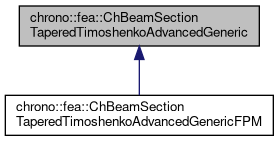## Description

Base class for all constitutive models of sections of Tapered Timoshenko beams.

This tapered section consists of two objects of ChBeamSectionTimoshenkoAdvancedGeneric. To be used with ChElementBeamTaperedTimoshenko.

#include <ChBeamSectionTaperedTimoshenko.h>[legend]

## Public Member Functions

void SetLength (double mv)
Set the length of beam element with two sections.

double GetLength () const
Get the length of beam element with two sections.

void SetLumpedMassMatrixType (bool mv)
Set the type of mass matrix: More...

bool GetLumpedMassMatrixType () const
Get the type of mass matrix： More...

void SetSectionA (std::shared_ptr< ChBeamSectionTimoshenkoAdvancedGeneric > my_material)
Set the section & material of beam element at end A.

void SetSectionB (std::shared_ptr< ChBeamSectionTimoshenkoAdvancedGeneric > my_material)
Set the section & material of beam element at end B.

Get the section & material of the element at end A.

Get the section & material of the element at end B.

virtual void ComputeInertiaMatrix (ChMatrixDynamic<> &M)
Compute the 12x12 sectional inertia matrix, as in {x_momentum,w_momentum}=[Mm]{xvel,wvel} The matrix is computed in the material reference (i.e. More...

virtual void ComputeInertiaDampingMatrix (ChMatrixNM< double, 12, 12 > &Ri, const ChVector<> &mW_A, const ChVector<> &mW_B)
Compute the 12x12 local inertial-damping (gyroscopic damping) matrix. More...

virtual void ComputeInertiaStiffnessMatrix (ChMatrixNM< double, 12, 12 > &Ki, const ChVector<> &mWvel_A, const ChVector<> &mWacc_A, const ChVector<> &mXacc_A, const ChVector<> &mWvel_B, const ChVector<> &mWacc_B, const ChVector<> &mXacc_B)
Compute the 12x12 local inertial-stiffness matrix. More...

virtual DampingCoefficients GetBeamRaleyghDamping () const
Get the average damping parameters of this tapered cross-section.

virtual void ComputeAverageSectionParameters ()
Compute the average section parameters: mass, inertia and rigidity, etc.

virtual std::shared_ptr< AverageSectionParametersGetAverageSectionParameters () const
Get the average sectional parameters(mass, inertia and rigidity, etc.) of this tapered cross-section.

## Public Attributes

bool compute_inertia_damping_matrix = true
Flag that turns on/off the computation of the [Ri] 'gyroscopic' inertial damping matrix. More...

bool compute_inertia_stiffness_matrix = true
Flag that turns on/off the computation of the [Ki] inertial stiffness matrix. More...

bool compute_Ri_Ki_by_num_diff = false
Flag for computing the Ri and Ki matrices via numerical differentiation even if an analytical expression is provided. More...

## Protected Member Functions

virtual void ComputeLumpedInertiaMatrix (ChMatrixNM< double, 12, 12 > &M)
Compute the 12x12 sectional inertia matrix in lumped format, as in {x_momentum,w_momentum}=[Mm]{xvel,wvel} The matrix is computed in the material reference (i.e. More...

virtual void ComputeSimpleConsistentInertiaMatrix (ChMatrixNM< double, 12, 12 > &M)

virtual void ComputeConsistentInertiaMatrix (ChMatrixNM< double, 12, 12 > &M)
A very generic implementation for consistent mass matrix, considering mass center offset and axis orientation. More...

## Protected Attributes

double length
The length of beam element with two sections.

The section & material of beam element at end A.

The section & material of beam element at end B.

bool use_lumped_mass_matrix
The type of mass matrix: More...

std::shared_ptr< AverageSectionParametersavg_sec_par

bool compute_ave_sec_par = false

## ◆ ComputeConsistentInertiaMatrix()

 void chrono::fea::ChBeamSectionTaperedTimoshenkoAdvancedGeneric::ComputeConsistentInertiaMatrix ( ChMatrixNM< double, 12, 12 > & M )
protectedvirtual

A very generic implementation for consistent mass matrix, considering mass center offset and axis orientation.

The transformation of mass center offset and mass principal axis orientation is hard-coded.

Parameters
 M 12x12 sectional mass matrix values here

 void chrono::fea::ChBeamSectionTaperedTimoshenkoAdvancedGeneric::ComputeInertiaDampingMatrix ( ChMatrixNM< double, 12, 12 > & Ri, const ChVector<> & mW_A, const ChVector<> & mW_B )
virtual

Compute the 12x12 local inertial-damping (gyroscopic damping) matrix.

The lumped format is used, need to multiple 0.5 * length to obtain the final inertial-damping matrix

Parameters
 Ri 12x12 sectional inertial-damping matrix values here mW_A current angular velocity of section of node A, in material frame mW_B current angular velocity of section of node B, in material frame

## ◆ ComputeInertiaMatrix()

 void chrono::fea::ChBeamSectionTaperedTimoshenkoAdvancedGeneric::ComputeInertiaMatrix ( ChMatrixDynamic<> & M )
virtual

Compute the 12x12 sectional inertia matrix, as in {x_momentum,w_momentum}=[Mm]{xvel,wvel} The matrix is computed in the material reference (i.e.

it is the sectional mass matrix)

Parameters
 M 12x12 sectional mass matrix values here

## ◆ ComputeInertiaStiffnessMatrix()

 void chrono::fea::ChBeamSectionTaperedTimoshenkoAdvancedGeneric::ComputeInertiaStiffnessMatrix ( ChMatrixNM< double, 12, 12 > & Ki, const ChVector<> & mWvel_A, const ChVector<> & mWacc_A, const ChVector<> & mXacc_A, const ChVector<> & mWvel_B, const ChVector<> & mWacc_B, const ChVector<> & mXacc_B )
virtual

Compute the 12x12 local inertial-stiffness matrix.

The lumped format is used, need to multiple 0.5 * length to obtain the final inertial-stiffness matrix

Parameters
 Ki 12x12 sectional inertial-stiffness matrix values here mWvel_A current angular velocity of section of node A, in material frame mWacc_A current angular acceleration of section of node A, in material frame mXacc_A current acceleration of section of node A, in material frame) mWvel_B current angular velocity of section of node B, in material frame mWacc_B current angular acceleration of section of node B, in material frame mXacc_B current acceleration of section of node B, in material frame

## ◆ ComputeLumpedInertiaMatrix()

 void chrono::fea::ChBeamSectionTaperedTimoshenkoAdvancedGeneric::ComputeLumpedInertiaMatrix ( ChMatrixNM< double, 12, 12 > & M )
protectedvirtual

Compute the 12x12 sectional inertia matrix in lumped format, as in {x_momentum,w_momentum}=[Mm]{xvel,wvel} The matrix is computed in the material reference (i.e.

it is the sectional mass matrix)

Parameters
 M 12x12 sectional mass matrix values here

## ◆ ComputeSimpleConsistentInertiaMatrix()

 void chrono::fea::ChBeamSectionTaperedTimoshenkoAdvancedGeneric::ComputeSimpleConsistentInertiaMatrix ( ChMatrixNM< double, 12, 12 > & M )
protectedvirtual
Parameters
 M 12x12 sectional mass matrix values here

## ◆ GetLumpedMassMatrixType()

inline

Get the type of mass matrix：

• true: lumped mass matrix.
• false: consistent mass matrix.

## ◆ SetLumpedMassMatrixType()

 void chrono::fea::ChBeamSectionTaperedTimoshenkoAdvancedGeneric::SetLumpedMassMatrixType ( bool mv )
inline

Set the type of mass matrix:

• true: lumped mass matrix, which is default.
• false: consistent mass matrix.

## ◆ compute_inertia_damping_matrix

Flag that turns on/off the computation of the [Ri] 'gyroscopic' inertial damping matrix.

If false, Ri=0. Can be used for cpu speedup, profiling, tests. Default: true.

## ◆ compute_inertia_stiffness_matrix

Flag that turns on/off the computation of the [Ki] inertial stiffness matrix.

If false, Ki=0. Can be used for cpu speedup, profiling, tests. Default: true.

## ◆ compute_Ri_Ki_by_num_diff

Flag for computing the Ri and Ki matrices via numerical differentiation even if an analytical expression is provided.

Children calsses must take care of this. Default: false.